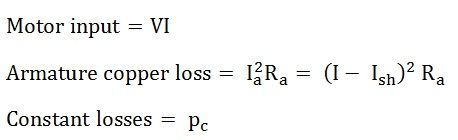# Swinburne’s Test

Swinburne’s Test is an indirect method of testing of DC machines. In this method, the losses are measured separately and the efficiency at any desired load is predetermined. Machines are tested for finding out losses, efficiency and temperature rise.

For small machines direct loading test is performed. For large shunt machines, indirect methods are used like Swinburne’s or Hopkinson’s test.

Contents:

The machine is running as a motor at rated voltage and speed. The connection diagram for DC shunt machine is shown in the figure below:

Ish is the shunt field current,

Therefore, no load armature current is given by the equation shown below:The no-load power input to the machine supplies the following, as given below:

• Iron loss in the core
• Friction losses in the bearings and commutators.
• Windage loss
• Armature copper loss at no load.

When the machine is loaded, the temperature of the armature winding and the field winding increases due to I2R losses.

For calculating I2R losses, hot resistances should be used. A stationary measurement of resistances at room temperature of t degree Celsius is made by passing a current through the armature and then field from a low voltage DC supply. Then the heated resistance, allowing a temperature rise of 50⁰C is found.

The equations are as follows:Where, α0 is the temperature coefficient of resistance at 0⁰C

Therefore,Stray loss = iron loss + friction loss + windage loss = input at no load – field copper loss – no load armature copper lossAlso, constant losses,If the constant losses of the machine are known, its efficiency at any other load can be determined as follows.

Let I be the load current at which efficiency is required.

### Efficiency when the machine is running as a Motor.Therefore, the total loss is given as:The efficiency of the motor is given below.

### Efficiency when the machine is running as a Generator.Therefore, the total loss is given as:The efficiency of the generator is given below:

The main advantages of Swinburne’s test are as follows:

• The power required to test a large machine is small. Thus, this method is an economical and convenient method of testing of DC machines.
• As the constant loss is known the efficiency can be predetermined at any load.